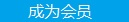您可以捐助，支持我们的公益事业。 1元 10元 50元 认证码：必填求知 文章 文库 Lib 视频 Code iProcess 课程 认证 咨询 工具 火云堂 讲座吧 成长之路要资料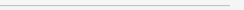订阅捐助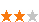61 次浏览     评价： 好 中 差
2019-3-15

 编辑推荐: 本文来自csdn，本文用的例子是脸部图像，但 DCGANs也可以被训练做其他类型的图像修复.

1. 我们首先要把图像解析成概率分布中的样本点

2. 这种解析让我们学习如何产生伪图像

3. 接着我们就能找到补全修复所需的最佳伪图像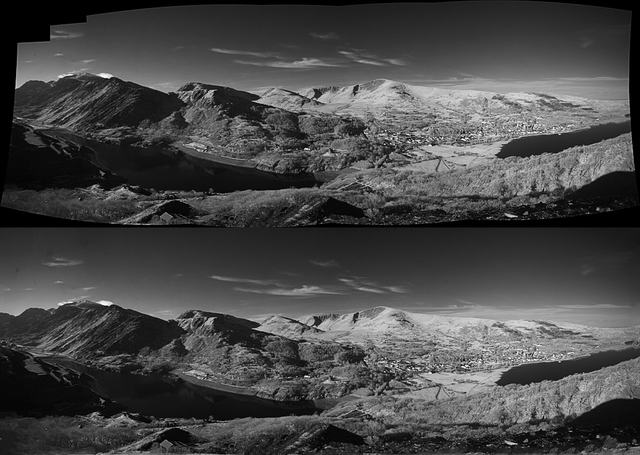1. 环境信息：你可以通过周围像素点的信息推断出缺失的像素点是什么。

2. 知觉信息：你会填补一些你认为是「正常」的内容，比如你在现实生活中或其他图像中可能看到的东西。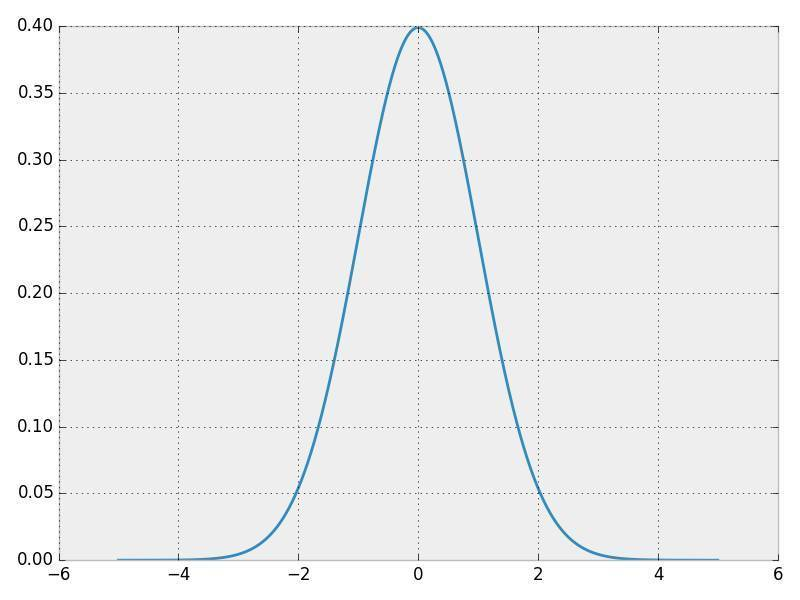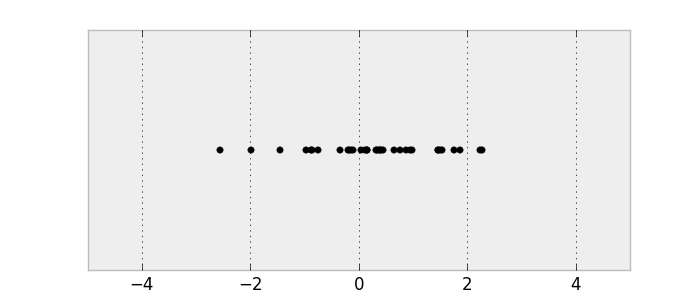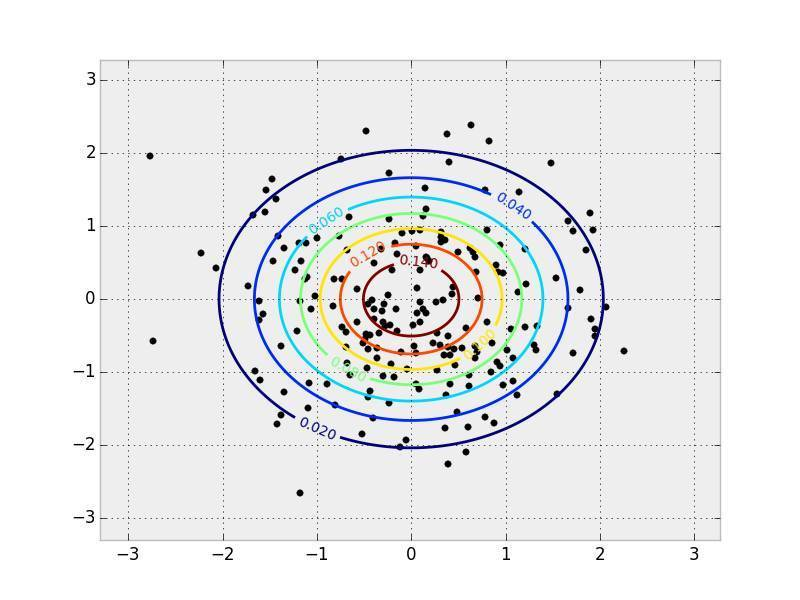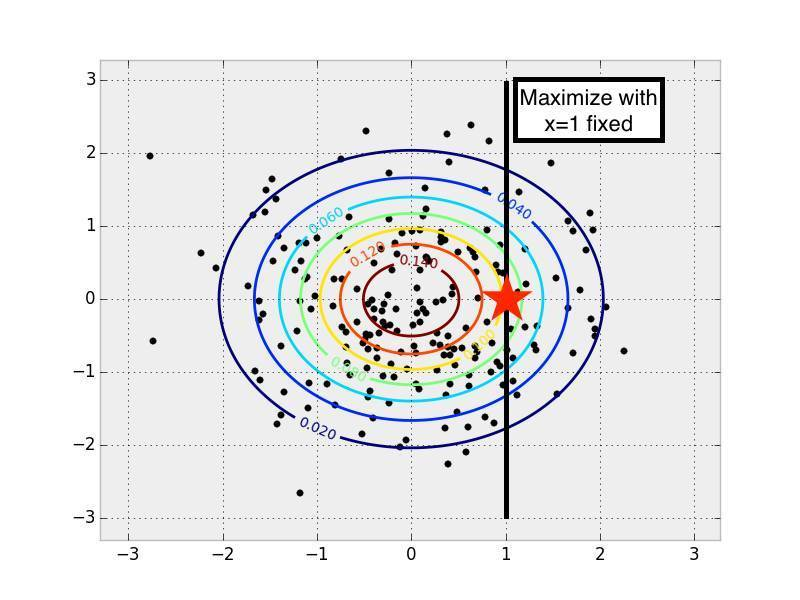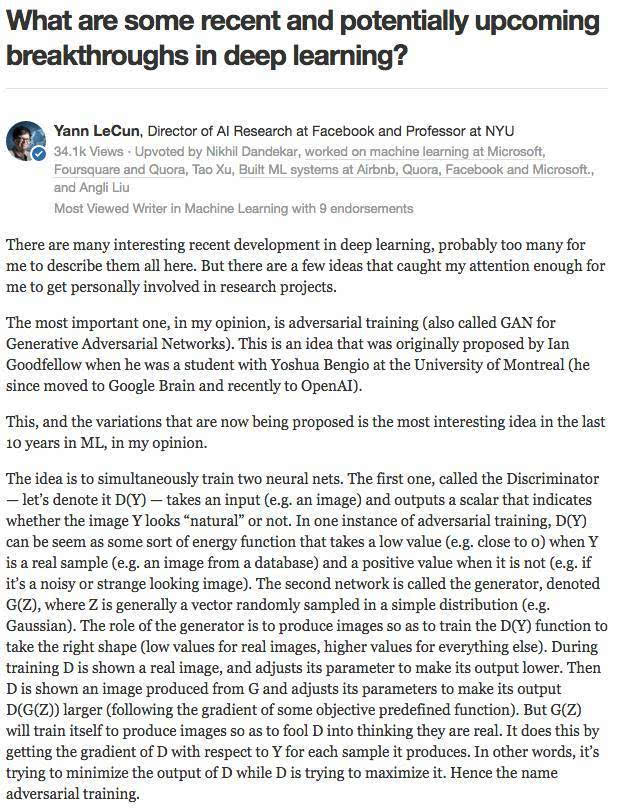EyeScream 博文中的对抗训练的街头霸王游戏类比（Street Fighter analogy for adversarial networks）。（博文地址：）这些网络互相争斗并且一起发展进步，就像两个玩对战游戏的人类。

[ML-Heavy] 生成对抗网络（Generative Adversarial Net ，GAN）的架构

z = np.random.uniform(-1, 1, 5)array([ 0.77356483, 0.95258473, -0.18345086, 0.69224724, -0.34718733])

def G(z):

...

return imageSamplez = np.random.uniform(-1, 1, 5)imageSample = G(z)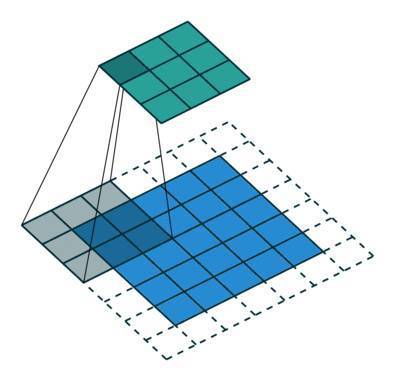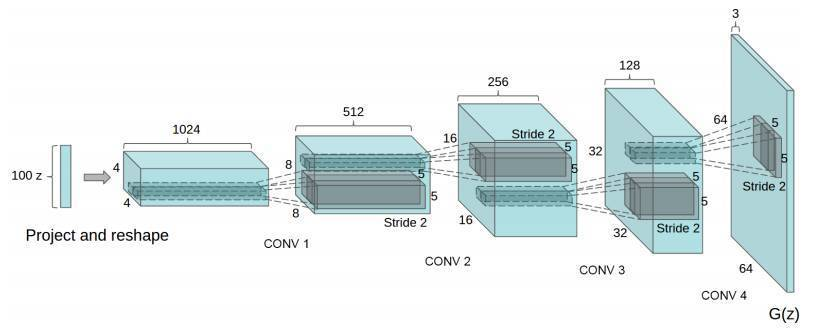DCGAN 论文还展现了其他的技巧，还有对 DCGAN 训练的调节方法，比如用批归一化（batch normalization）或 leaky ReLU。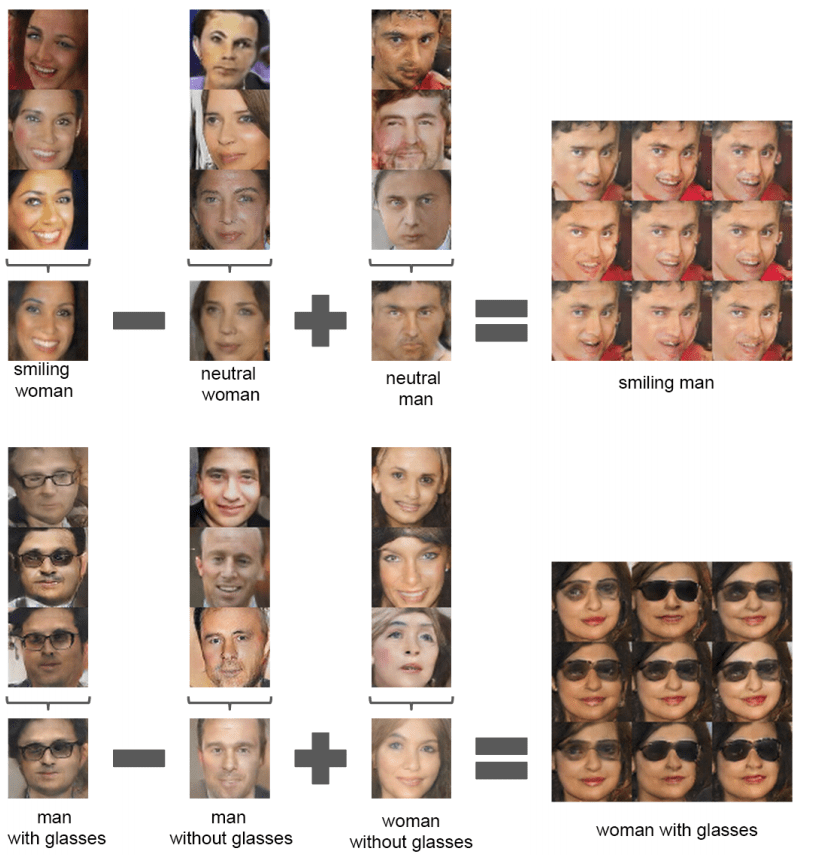[ML-Heavy]训练DCGANs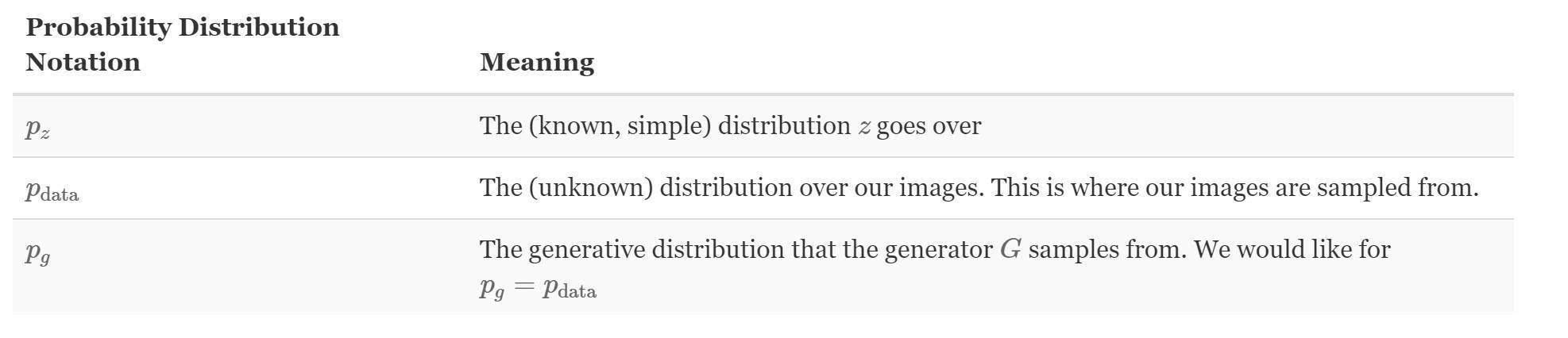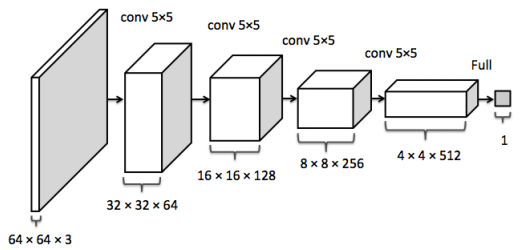1. 对于真实数据分布 x~ pdata中的每个图像最大化 D(x)。

2. 对于来自非真实数据分布 x? pdata 的每个图像把 D(x) 最小化。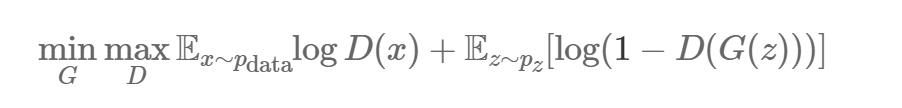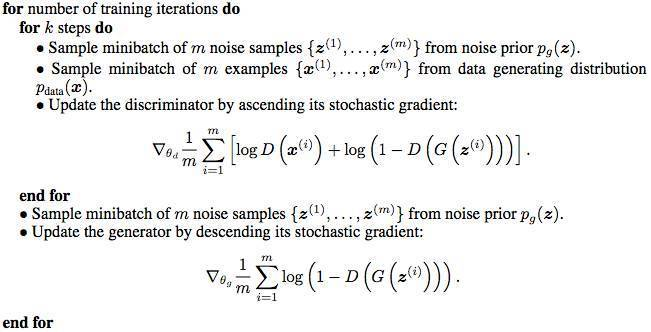GAN paper里的GAN训练算法

GitHub 上有很多好的 GAN 和 DCGAN 实现方法：

goodfeli/adversarial : GAN 论文作者放出的 Theano GAN 实现方法。tqchen/mxnet-gan : 非正式MXNet GAN实现。

Newmu/dcgan_code : DCGAN 论文作者放出的Theano DCGAN 实现方法。

soumith/dcgan.torch: DCGAN 论文作者之一（Soumith Chintala）放出的Torch DCGAN 实现方法。

carpedm20/DCGAN-tensorflow : 非正式 TensorFlow DCGANU 实现。

openai/improved-gan: OpenAI第一篇论文背后的代码。大幅修正了carpedm20/DCGAN-tensorflow。

mattya/chainer-DCGAN :非正式 Chainer DCGAN 实现。

jacobgil/keras-dcgan: 非正式（也不完整）Keras DCGAN 实现。

[ML-Heavy]TensorFlow上的 DCGANs

 def generator(self, z, y=None): 　　self.z_, self.h0_w, self.h0_b = linear(z, self.gf_dim*8*4*4, 　　'g_h0_lin', with_w=True) 　　self.h0 = tf.reshape(self.z_, [-1, 4, 4, self.gf_dim * 8]) 　　h0 = tf.nn.relu(self.g_bn0(self.h0)) 　　self.h1, self.h1_w, self.h1_b = conv2d_transpose(h0, 　　[self.batch_size, 8, 8, self.gf_dim*4], name='g_h1', with_w=True) 　　h1 = tf.nn.relu(self.g_bn1(self.h1)) 　　h2, self.h2_w, self.h2_b = conv2d_transpose(h1, 　　[self.batch_size, 16, 16, self.gf_dim*2], name='g_h2', with_w=True) 　　h2 = tf.nn.relu(self.g_bn2(h2)) 　　h3, self.h3_w, self.h3_b = conv2d_transpose(h2, 　　[self.batch_size, 32, 32, self.gf_dim*1], name='g_h3', with_w=True) 　　h3 = tf.nn.relu(self.g_bn3(h3)) 　　h4, self.h4_w, self.h4_b = conv2d_transpose(h3, 　　[self.batch_size, 64, 64, 3], name='g_h4', with_w=True) 　　return tf.nn.tanh(h4) def discriminator(self, image, reuse=False, y=None): 　　if reuse: 　　tf.get_variable_scope().reuse_variables() 　　h0 = lrelu(conv2d(image, self.df_dim, name='d_h0_conv')) 　　h1 = lrelu(self.d_bn1(conv2d(h0, self.df_dim*2, name='d_h1_conv'))) 　　h2 = lrelu(self.d_bn2(conv2d(h1, self.df_dim*4, name='d_h2_conv'))) 　　h3 = lrelu(self.d_bn3(conv2d(h2, self.df_dim*8, name='d_h3_conv'))) 　　h4 = linear(tf.reshape(h3, [-1, 8192]), 1, 'd_h3_lin') 　　return tf.nn.sigmoid(h4), h4

 self.G = self.generator(self.z)self.D, self.D_logits = self.discriminator(self.images)self.D_, self.D_logits_ = self.discriminator(self.G, reuse=True)

 self.d_loss_real = tf.reduce_mean( 　　tf.nn.sigmoid_cross_entropy_with_logits(self.D_logits, 　　tf.ones_like(self.D)))self.d_loss_fake = tf.reduce_mean( 　　tf.nn.sigmoid_cross_entropy_with_logits(self.D_logits_, 　　tf.zeros_like(self.D_)))self.d_loss = self.d_loss_real + self.d_loss_fakeself.g_loss = tf.reduce_mean( 　　tf.nn.sigmoid_cross_entropy_with_logits(self.D_logits_, 　　tf.ones_like(self.D_)))

 t_vars = tf.trainable_variables()self.d_vars = [var for var in t_vars if 'd_' in var.name]self.g_vars = [var for var in t_vars if 'g_' in var.name]

 d_optim = tf.train.AdamOptimizer(config.learning_rate, beta1=config.beta1) \ 　　.minimize(self.d_loss, var_list=self.d_vars)g_optim = tf.train.AdamOptimizer(config.learning_rate, beta1=config.beta1) \ 　　.minimize(self.g_loss, var_list=self.g_vars)

 for epoch in xrange(config.epoch): 　　... 　　for idx in xrange(0, batch_idxs): 　　batch_images = ... 　　batch_z = np.random.uniform(-1, 1, [config.batch_size, self.z_dim]) \ 　　.astype(np.float32) 　　# Update D network 　　＃更新一个 D 网络 　　_, summary_str = self.sess.run([d_optim, self.d_sum], 　　feed_dict={ self.images: batch_images, self.z: batch_z }) 　　# Update G network 　　＃更新一个 G 网络 　　_, summary_str = self.sess.run([g_optim, self.g_sum], 　　feed_dict={ self.z: batch_z }) 　　# Run g_optim twice to make sure that d_loss does not go to zero* 　　# (different from paper) 　　＃运行两次*g_optim 以确保 d_loss 不会变成0 　　＃（与论文里不一样） 　　_, summary_str = self.sess.run([g_optim, self.g_sum], 　　feed_dict={ self.z: batch_z }) 　　errD_fake = self.d_loss_fake.eval({self.z: batch_z}) 　　errD_real = self.d_loss_real.eval({self.images: batch_images}) 　　errG = self.g_loss.eval({self.z: batch_z})

 git clone https://github.com/cmusatyalab/openface.git git clone https://github.com/bamos/dcgan-completion.tensorflow.git

 cd openface pip2 install -r requirements.txt 　python2 setup.py installcd ..

 ./openface/util/align-dlib.py data/dcgan-completion.tensorflow/data/your-dataset/raw align innerEyesAndBottomLip data/dcgan-completion.tensorflow/data/your-dataset/aligned --size 64

 cd dcgan-completion.tensorflow/data/your-dataset/aligned bfind . -name ' .png' -exec mv {} . \; 　find . -type d -empty -deletecd ../../..

 ./train-dcgan.py --dataset ./data/your -dataset/aligned --epoch 20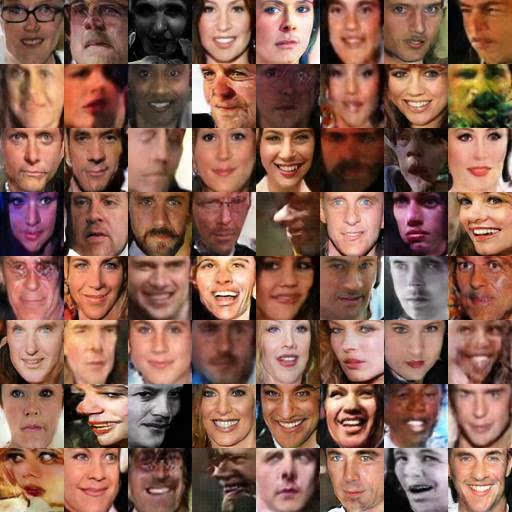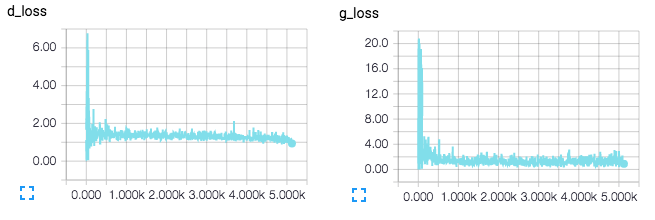TensorBoard 损耗的可视化，将在训练中实时更新。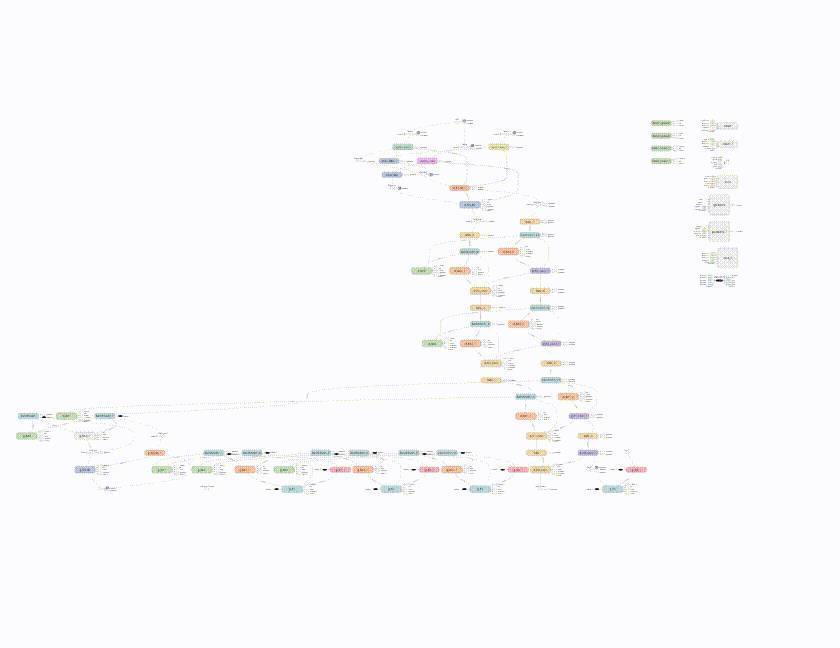DCGAN 网络的 TensorBoard 可视化。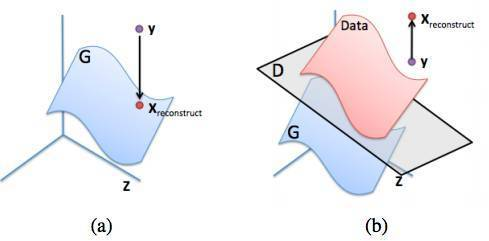(a): y 在生成分布（蓝色）上的理想重建

(b): 试图只通过最大化 D(y) 重建 y 的失败例子。图像来源：inpainting paper

[ML-Heavy] pg上映射的损失函数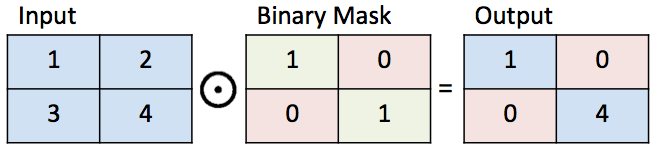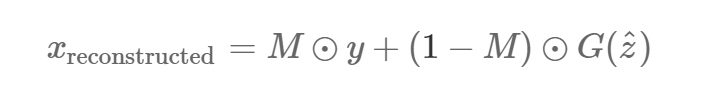||x||1=∑i|xi|||x||1=∑i|xi| 是某些向量 x 的 ?t 标准。 ?2 标准是另一个合理的选择，但那篇图像修复论文说 ?1 标准在实际应用中效果更好。在这个理想的情况下，所有已知位置的像素点在 y 和 G(z) 之间都是一样的。所以对已知像素点

G(z)i?yi= 0，因而Lcontextual(z)=0。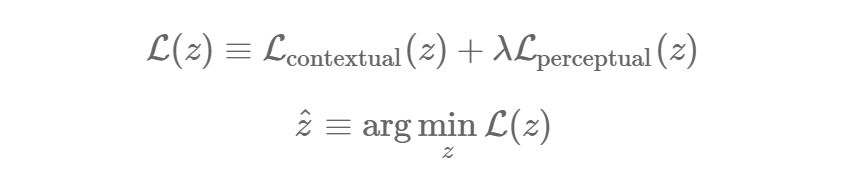λ 是一个控制输入环节缺失如何与直觉缺失相联系的超参数。（我用了默认的 λ=0.1，而且还没有太多改变它。）接着就跟前面一样，重构后的图像用 y （G(z? )）填补了缺失值：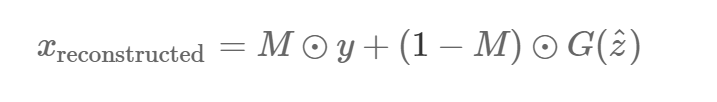self.mask = tf.placeholder(tf.float32, [None] + self.image_shape, name='mask')

 self.contextual_loss = tf.reduce_sum( tf.contrib.layers.flatten( 　tf.abs(tf.mul(self.mask, self.G) - tf.mul(self.mask, self.images))), 1)self.perceptual_loss = self.g_lossself.complete_loss = self.contextual_loss + self.lam*self.perceptual_lossself.grad_complete_loss = tf.gradients(self.complete_loss, self.z)

 if config.maskType == 'center': 　　scale = 0.25 　　asser (scale <= 0.5) 　　mask = np.ones(self.image_shape) 　　sz = self.image_size 　　l = int(self.image_size*scale) 　　u = int(self.image_size*(1.0-scale)) 　　mask[l:u, l:u, :] = 0.0

 for idx in xrange(0, batch_idxs): 　　batch_images = ... 　　batch_mask = np.resize(mask, [self.batch_size] + self.image_shape) 　　zhats = np.random.uniform(-1, 1, size=(self.batch_size, self.z_dim)) 　　v = 0 　　for i in xrange(config.nIter): 　　fd = { 　　self.z: zhats, 　　self.mask: batch_mask, 　　self.images: batch_images, 　　} 　　run = [self.complete_loss, self.grad_complete_loss, self.G] 　　loss, g, G_imgs = self.sess.run(run, feed_dict=fd) 　　v_prev = np.copy(v) 　　v = config.momentum v - config.lrg 　　zhats += -config.momentum v_prev + (1+config.momentum)*v 　　np.clip(zhats, -1, 1)

 ./complete.py ./data/your-test-data/aligned/***** --outDir outputImages

 cd outputImages 　convert -delay 10 -loop 0 before.png completed/ .png completion.gif

1. 把图像解析成一个概率分布中的样本点

2. 生成伪图像

3. 找到修复所需的最佳伪图像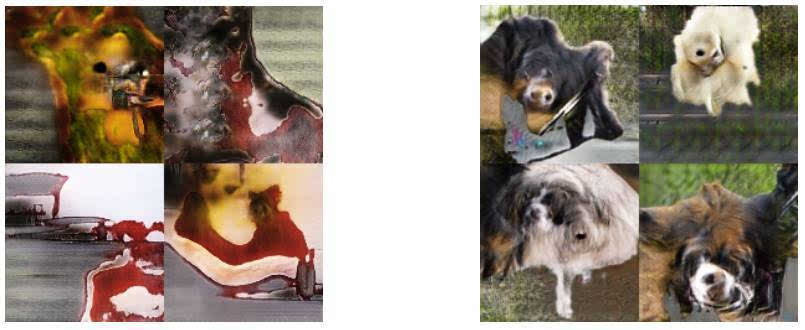ImageNet 上的 DCGAN 样本（左）和改进后的 GAN 样本（右，本文中为涉及到），表明我们还没有理解如何对每种类型图像运用 GAN 。图像来源：improved GAN paper61 次浏览  评价: 好 中 差订阅捐助
 相关文章 我们该如何设计数据库 数据库设计经验谈 数据库设计过程 数据库编程总结
 相关文档 数据库性能调优技巧 数据库性能调整 数据库性能优化讲座 数据库系统性能调优系列
 相关课程 高性能数据库设计与优化 高级数据库架构师 数据仓库和数据挖掘技术 Hadoop原理、部署与性能调优每天2个文档/视频 扫描微信二维码订阅
 订阅技术月刊 获得每月300个技术资源